# Quantum state

In quantum physics, quantum state refers to the state of a quantum system. A quantum state is given as a vector in a Hilbert space, called the state vector. For example, when dealing with the energy spectrum of the electron in a hydrogen atom, the relevant state vector is identified by the principal quantum number${ n }$. For a more complicated case, consider Bohm’s formulation of the EPR experiment, where the state vector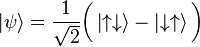$left|psirightrang = frac{1}{sqrt{2}}bigg(left|uparrowdownarrowrightrang - left|downarrowuparrowrightrang bigg)$

involves superposition of joint spin states for two particles.

More generally, a quantum state can be either pure or mixed. The above example is pure. Mathematically, a pure quantum state is represented by a state vector in a Hilbert space over complex numbers, which is a generalization of our more usual three-dimensional space. If this Hilbert space is represented as a function space, then its elements are called wave functions.

A mixed quantum state corresponds to a probabilistic mixture of pure states; however, different distributions of pure states can generate equivalent (i.e., physically indistinguishable) mixed states. Quantum states, mixed as well as pure, are described by so-called density matrices.

For example, if the spin of an electron is measured in any direction, e.g. with a Stern–Gerlach experiment, there are two possible results: up or down. The Hilbert space for the electron’s spin is therefore two-dimensional. A pure state here is represented by a two-dimensionalcomplex vector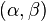$(alpha, beta)$, with a length of one; that is, with$|alpha|^2 + |beta|^2 = 1,$

where$|alpha|$ and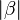$|beta|$ are the absolute values of$alpha$ and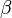$beta$. A mixed state, in this case, is a$2 times 2$ matrix that is Hermitian, positive-definite, and has trace 1.

Before a particular measurement is performed on a quantum system, the theory usually gives only a probability distribution for the outcome, and the form that this distribution takes is completely determined by the quantum state and the observable describing the measurement. These probability distributions arise for both mixed states and pure states: it is impossible in quantum mechanics (unlike classical mechanics) to prepare a state in which all properties of the system are fixed and certain. This is exemplified by theuncertainty principle, and reflects a core difference between classical and quantum physics. Even in quantum theory, however, for every observable there are states that determine its value exactly.

## Conceptual description

### Pure statesProbability densities for the electron of a hydrogen atom in different quantum states.

In the mathematical formulation of quantum mechanics, pure quantum states correspond to vectorsin a Hilbert space, while each observable quantity (such as the energy or momentum of a particle) is associated with a mathematical operator. The operator serves as a linear function which acts on the states of the system. The eigenvalues of the operator correspond to the possible values of the observable, i.e. it is possible to observe a particle with a momentum of 1 kg⋅m/s if and only if one of the eigenvalues of the momentum operator is 1 kg⋅m/s. The corresponding eigenvector (which physicists call an “eigenstate”) with eigenvalue 1 kg⋅m/s would be a quantum state with a definite, well-defined value of momentum of 1 kg⋅m/s, with no quantum uncertainty. If its momentum were measured, the result is guaranteed to be 1 kg⋅m/s.

On the other hand, a system in a linear combination of multiple different eigenstates does in general have quantum uncertainty. We can represent this linear combination of eigenstates as: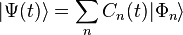$|Psi(t)rangle = sum_n C_n(t) |Phi_nrang$.

The coefficient which corresponds to a particular state in the linear combination is complex thus allowing interference effects between states. The coefficients are time dependent. How a quantum system changes in time is governed by the time evolution operator. The symbols “|” and ““surrounding the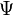$Psi$ are part of bra–ket notation.

Statistical mixtures of states are separate from a linear combination. A statistical mixture of states occurs with a statistical ensemble of independent systems. Statistical mixtures represent the degree of knowledge whilst the uncertainty within quantum mechanics is fundamental. Mathematically, a statistical mixture is not a combination of complex coefficients but rather a combination of probabilities of different states$Phi_n$.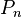$P_n$ represents the probability of a randomly selected system being in the state$Phi_n$. Unlike the linear combination case each system is in a definite eigenstate.

In general we must understand the expectation value$langle A rangle _sigma$ of an observable A as a statistical mean. It is this mean and the distribution of probabilities that is predicted by physical theories.

There is no state which is simultaneously an eigenstate for all observables. For example, we cannot prepare a state such that both the position measurement Q(t) and the momentum measurement P(t) (at the same time t) are known exactly; at least one of them will have a range of possible values. This is the content of the Heisenberg uncertainty relation.

Moreover, in contrast to classical mechanics, it is unavoidable that performing a measurement on the system generally changes its state. More precisely: After measuring an observable A, the system will be in an eigenstate of A; thus the state has changed, unless the system was already in that eigenstate. This expresses a kind of logical consistency: If we measure A twice in the same run of the experiment, the measurements being directly consecutive in time, then they will produce the same results. This has some strange consequences however:

Consider two observables, A and B, where A corresponds to a measurement earlier in time than B. Suppose that the system is in an eigenstate of B. If we measure only B, we will not notice statistical behaviour. If we measure first A and then B in the same run of the experiment, the system will transfer to an eigenstate of A after the first measurement, and we will generally notice that the results of B are statistical. Thus: Quantum mechanical measurements influence one another, and it is important in which order they are performed.

Another feature of quantum states becomes relevant if we consider a physical system that consists of multiple subsystems; for example, an experiment with two particles rather than one. Quantum physics allows for certain states, called entangled states, that show certain statistical correlations between measurements on the two particles which cannot be explained by classical theory. For details, see entanglement. These entangled states lead to experimentally testable properties (Bell’s theorem) that allow us to distinguish between quantum theory and alternative classical (non-quantum) models.

### Schrödinger picture vs. Heisenberg picture

In the discussion above, we have taken the observables P(t), Q(t) to be dependent on time, while the state σ was fixed once at the beginning of the experiment. This approach is called the Heisenberg picture. One can, equivalently, treat the observables as fixed, while the state of the system depends on time; that is known as the Schrödinger picture. Conceptually (and mathematically), both approaches are equivalent; choosing one of them is a matter of convention.

Both viewpoints are used in quantum theory. While non-relativistic quantum mechanics is usually formulated in terms of the Schrödinger picture, the Heisenberg picture is often preferred in a relativistic context, that is, for quantum field theory. Compare with Dirac picture.

## Formalism in quantum physics

### Pure states as rays in a Hilbert space

Quantum physics is most commonly formulated in terms of linear algebra, as follows. Any given system is identified with some finite- or infinite-dimensional Hilbert space. The pure states correspond to vectors of norm 1. Thus the set of all pure states corresponds to the unit sphere in the Hilbert space.

If two unit vectors differ only by a scalar of magnitude 1, known as a “global phase factor”, then they are indistinguishable. Therefore, distinct pure states can be put in correspondence with “rays” in the Hilbert space, or equivalently points in the projective Hilbert space.

### Bra–ket notation

Calculations in quantum mechanics make frequent use of linear operators, inner products, dual spaces and Hermitian conjugation. In order to make such calculations more straightforward, and to obviate the need (in some contexts) to fully understand the underlying linear algebra, Paul Dirac invented a notation to describe quantum states, known as bra-ket notation. Although the details of this are beyond the scope of this article (see the article bra–ket notation), some consequences of this are:

• The variable name used to denote a vector (which corresponds to a pure quantum state) is chosen to be of the form$|psirangle$ (where the “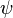$psi$” can be replaced by any other symbols, letters, numbers, or even words). This can be contrasted with the usual mathematical notation, where vectors are usually bold, lower-case letters, or letters with arrows on top.
• Instead of vector, the term ket is used synonymously.
• Each ket$|psirangle$ is uniquely associated with a so-called bra, denoted$langlepsi|$, which is also said to correspond to the same physical quantum state. Technically, the bra is theadjoint of the ket. It is an element of the dual space, and related to the ket by the Riesz representation theorem. In a finite-dimensional space with a chosen basis, writing$|psirangle$ as a column vector,$langlepsi|$ is a row vector; just take the transpose and entry-wise complex conjugate of$|psirangle$.
• Inner products (also called brackets) are written so as to look like a bra and ket next to each other:$lang psi_1|psi_2rang$. (The phrase “bra-ket” is supposed to resemble “bracket”.)

### Spin

The angular momentum has the same dimension as the Planck constant and, at quantum scale, behaves as a discrete degree of freedom. Most particles possess a kind of intrinsic angular momentum that does not appear at all in classical mechanics and arises from Dirac’s relativistic generalization of the theory. Mathematically it is described withspinors. In non-relativistic quantum mechanics the fundamental representations of SU(2) are used to describe this additional freedom. For a given particle, it is characterized quantitatively by a non-negative number S that, in units of Planck’s reduced constant ħ, is either an integer (0, 1, 2 …) or a half-integer (1/2, 3/2, 5/2 …). For a massive particle of the spin S, its spin quantum number m always assumes 2S + 1 possible values from the set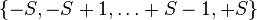${ -S, -S+1, ldots +S-1, +S }$

As a consequence, the quantum state of a particle is described by a vector-valued wave function with values in C2S+1 or, equivalently, by a complex-valued function of four variables: one discrete quantum number variable is added to three continuous (spatial) variables.

### Many-body states and particle statistics

The quantum state of a system of N particles is described by a complex-valued function with four variables per particle, e.g.$|psi (mathbf r_1,m_1;dots ;mathbf r_N,m_N)rangle.$

Here, the spin variables mν assume values from the set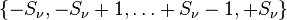${ -S_nu, -S_nu +1, ldots +S_nu -1,+S_nu }$

where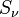$S_nu$ is the spin of νth particle.

Moreover, the case of identical particles makes the difference between bosons (particles with integer spin) and fermions (particles with half-integer spin). The above N-particle function must either be symmetrized (in the bosonic case) or anti-symmetrized (in the fermionic case) with respect to the particle numbers. If not all N particles are identical, but some of them are, then the function must be (anti)symmetrized over respective groups of variables, for each flavour of particles separately according to its statistics.

Electrons are fermions with S = 1/2, photons (quanta of light) are bosons with S = 1 (although in the vacuum they are massless and can’t be described with Schrödingerian mechanics).

Apart from the symmetrization or anti-symmetrization, N-particle spaces of states can thus simply be obtained by tensor products of one-particle spaces, to which we return herewith.

### Basis states of one-particle systems

As with any Hilbert space, if a basis is chosen for the Hilbert space of a system, then any ket can be expanded as a linear combination of those basis elements. Symbolically, given basis kets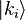$|{k_i}rang$, any ket$|psirang$ can be written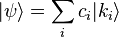$| psi rang = sum_i c_i |{k_i}rangle$

where ci are complex numbers. In physical terms, this is described by saying that$|psirang$ has been expressed as a quantum superposition of the states$|{k_i}rang$. If the basis kets are chosen to be orthonormal (as is often the case), then$c_i=lang {k_i} | psi rang$.

One property worth noting is that the normalized states$|psirang$ are characterized by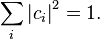$sum_i left | c_i right | ^2 = 1.$

Expansions of this sort play an important role in measurement in quantum mechanics. In particular, if the$|{k_i}rang$ are eigenstates (with eigenvalues ki) of an observable, and that observable is measured on the normalized state$|psirang$, then the probability that the result of the measurement is ki is |ci|2. (The normalization condition above mandates that the total sum of probabilities is equal to one.)

A particularly important example is the position basis, which is the basis consisting of eigenstates of the observable which corresponds to measuring position. If these eigenstates are nondegenerate (for example, if the system is a single, spinless particle), then any ket$|psirang$ is associated with a complex-valued function of three-dimensional space: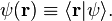$psi(mathbf{r}) equiv lang mathbf{r} | psi rang.$

This function is called the wavefunction corresponding to$|psirang$.

### Superposition of pure states

One aspect of quantum states, mentioned above, is that superpositions of them can be formed. If$|alpharangle$ and$|betarangle$ are two kets corresponding to quantum states, the ket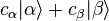$c_alpha|alpharang+c_beta|betarang$

is a different quantum state (possibly not normalized). Note that which quantum state it is depends on both the amplitudes and phases (arguments) of$c_alpha$ and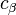$c_beta$. In other words, for example, even though$|psirang$ and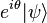$e^{itheta}|psirang$ (for real θ) correspond to the same physical quantum state, they are not interchangeable, since for example$|phirang+|psirang$ and$|phirang+e^{itheta}|psirang$ do not (in general) correspond to the same physical state. However,$|phirang+|psirang$ and$e^{itheta}(|phirang+|psirang)$ do correspond to the same physical state. This is sometimes described by saying that “global” phase factors are unphysical, but “relative” phase factors are physical and important.

One example of a quantum interference phenomenon that arises from superposition is the double-slit experiment. The photon state is a superposition of two different states, one of which corresponds to the photon having passed through the left slit, and the other corresponding to passage through the right slit. The relative phase of those two states has a value which depends on the distance from each of the two slits. Depending on what that phase is, the interference is constructive at some locations and destructive in others, creating the interference pattern. By the analogy with coherence in other wave phenomena, a superposed state can be referred to as a coherent superposition.

Another example of the importance of relative phase in quantum superposition is Rabi oscillations, where the relative phase of two states varies in time due to the Schrödinger equation. The resulting superposition ends up oscillating back and forth between two different states.

### Mixed states

A pure quantum state is a state which can be described by a single ket vector, as described above. A mixed quantum state is a statistical ensemble of pure states (see quantum statistical mechanics). Mixed states inevitably arise from pure states when, for a composite quantum system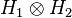$H_1 otimes H_2$ with an entangled state on it, the part$H_2$ is inaccessible to the observer. The state of the part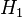$H_1$ is expressed then as the partial trace over$H_2$.

A mixed state cannot be described as a ket vector. Instead, it is described by its associated density matrix (or density operator), usually denoted ρ. Note that density matrices can describe both mixed and pure states, treating them on the same footing. Moreover, a mixed quantum state on a given quantum system described by a Hilbert space$H$ can be always represented as the partial trace of a pure quantum state (called a purification) on a larger bipartite system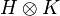$H otimes K$ for a sufficiently large Hilbert space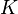$K$.

The density matrix is defined as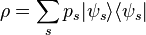$rho = sum_s p_s | psi_s rangle langle psi_s |$

where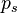$p_s$ is the fraction of the ensemble in each pure state$|psi_srangle.$ Here, one typically uses a one-particle formalism to describe the average behaviour of an N-particle system.

A simple criterion for checking whether a density matrix is describing a pure or mixed state is that the trace of ρ2 is equal to 1 if the state is pure, and less than 1 if the state is mixed. Another, equivalent, criterion is that the von Neumann entropy is 0 for a pure state, and strictly positive for a mixed state.

The rules for measurement in quantum mechanics are particularly simple to state in terms of density matrices. For example, the ensemble average (expectation value) of a measurement corresponding to an observable A is given by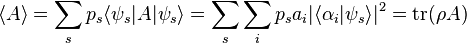$langle A rangle = sum_s p_s langle psi_s | A | psi_s rangle = sum_s sum_i p_s a_i | langle alpha_i | psi_s rangle |^2 = operatorname{tr}(rho A)$

where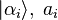$|alpha_irangle, ; a_i$ are eigenkets and eigenvalues, respectively, for the operator A, and “tr” denotes trace. It is important to note that two types of averaging are occurring, one being a weighted quantum superposition over the basis kets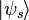$|psi_srangle$ of the pure states, and the other being a statistical (said incoherent) average with the probabilities ps of those states.

## Interpretation

Although theoretically, for a given quantum system, a state vector provides the full information about its evolution, it is not easy to understand what information about the “real world” does it carry. Due to the uncertainty principle, a state, even if has the value of one observable exactly defined (i.e. the observable has this state as an eigenstate), cannot exactly define values of all observables.

For state vectors (pure states), probability amplitudes offer a probabilistic interpretation. It can be generalized for all states (including mixed), for instance, as expectation values mentioned above.

## Mathematical generalizations

States can be formulated in terms of observables, not of a vector space. These are positive normalized linear functionals on a C*-algebra, or sometimes other classes of algebras of observables. See State on a C*-algebra and Gelfand–Naimark–Segal construction for more details.

Source: Wikipedia and RCAS library